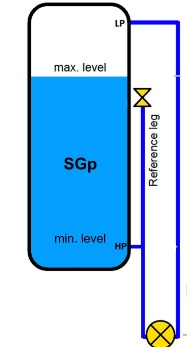# Zero Elevation and Zero Suppression in Level Measurement### Introduction

It would be idealistic to say that the DP cell can always be located at the exact the bottom of the vessel we are measuring fluid level in. Hence, the measuring system has to consider the hydrostatic pressure of the fluid in the sensing lines themselves. This leads to two compensations required.Zero Elevation and Zero Suppression in Level Measurement.

### Zero Suppression

In some cases, it is not possible to mount the level transmitter right at the base level of the tank. Say for maintenance purposes, the level transmitter has to be mounted X meters below the base of an open tank as shown in FigureThe liquid in the tank exerts a varying pressure that is proportional to its level H on the high-pressure side of the transmitter. The liquid in the high pressure impulse line also exerts a pressure on the high-pressure side.

However, this pressure is a constant (P = S⋅ X) and is present at all times.

When the liquid level is at H meters, pressure on the high-pressure side of the transmitter will be:

Phigh = S⋅H + S⋅ X + Patm                              s=specific gravity of liquid.

Plow = Patm

ΔP = Phigh – Plow = S⋅H + S⋅ X

That is, the pressure on the high-pressure side is always higher than the actual pressure exerted by the liquid column in the tank (by a value of S⋅ X). This constant pressure would cause an output signal that is higher than 4 mA when the tank is empty and above 20 mA when it is full. The transmitter has to be negatively biased by a value of -S⋅ X so that the output of the transmitter is proportional to the tank level (S⋅H) only. This procedure is called Zero Suppression and it can be done during calibration of the transmitter. A zero suppression kit can be installed in the transmitter for this purpose.

### Zero Elevation

When a wet leg installation is used (see Figure below), the low-pressure side of the level transmitter will always experience a higher pressure than the high-pressure side. This is due to the fact that the height of the wet leg (X) is always equal to or greater than the maximum height of the liquid column (H) inside the tank.When the liquid level is at H meters, we have:

Phigh = Pgas + S⋅H

Plow = Pgas + S⋅ X

ΔP = Phigh – Plow = S⋅H – S⋅ X

= – S (X – H)

The differential pressure ΔP sensed by the transmitter is always a negative number (i.e., low pressure side is at a higher pressure than high pressure side). ΔP increases from P = -S⋅ X to P = -S (X-H) as the tank level rises from 0% to 100%

If the transmitter were not calibrated for this constant negative error (-S⋅ X), the transmitter output would read low at all times.

To properly calibrate the transmitter, a positive bias (+S⋅ X) is needed to elevate the transmitter output. This positive biasing technique is called zero elevation.

Scroll to Top